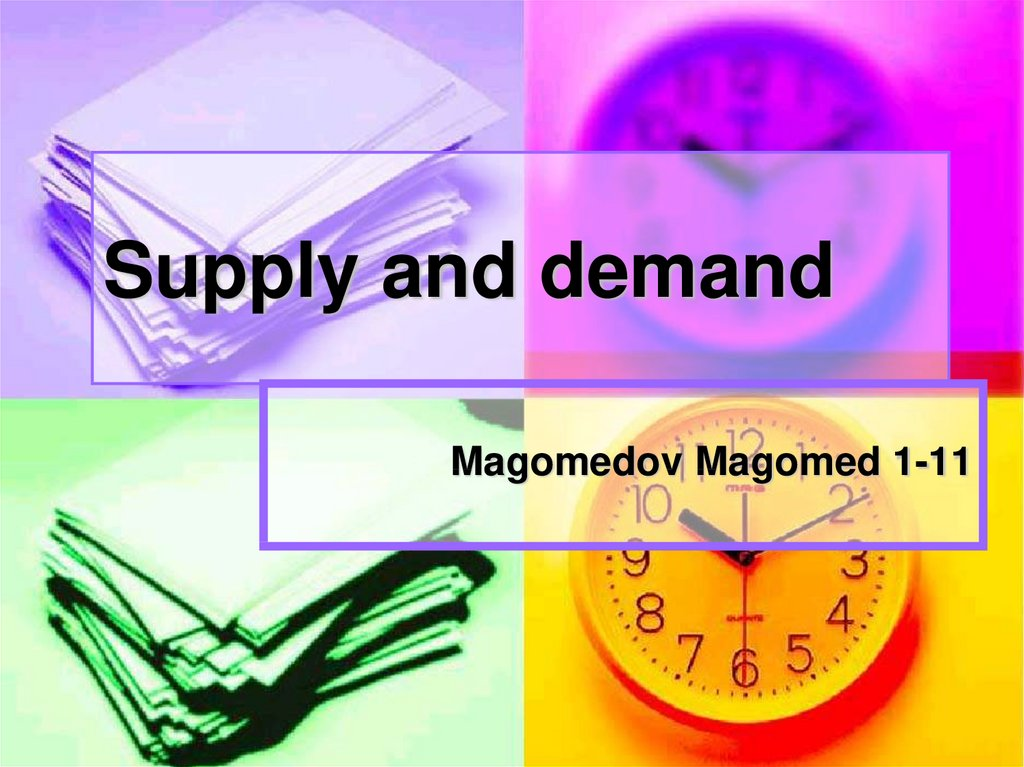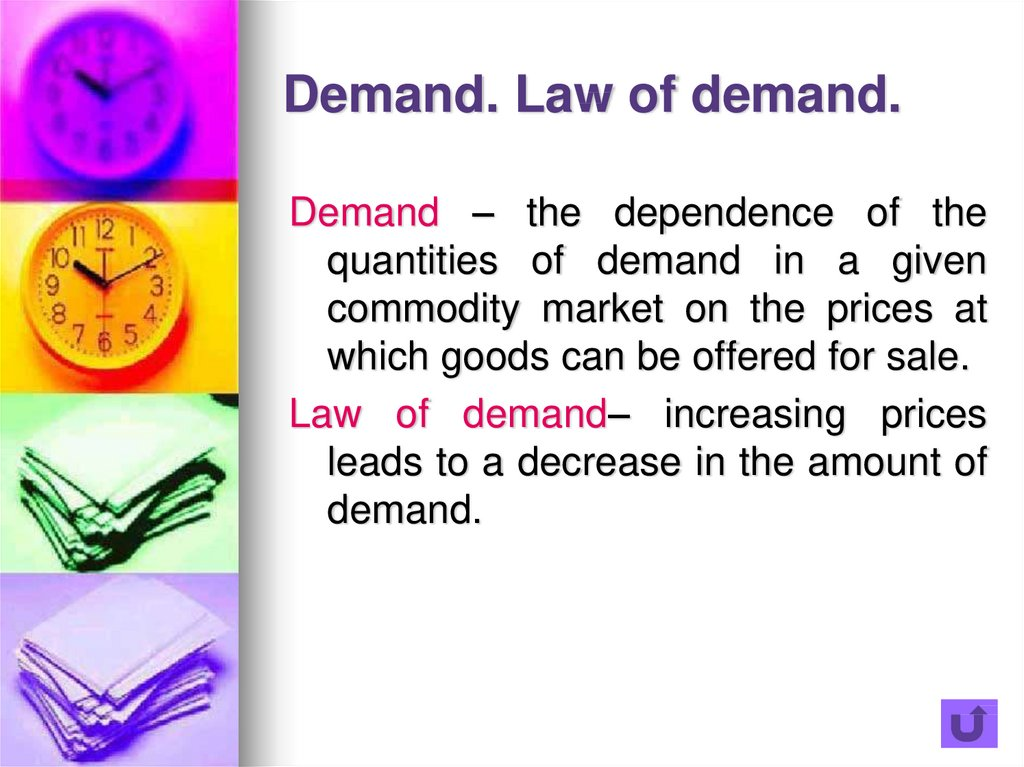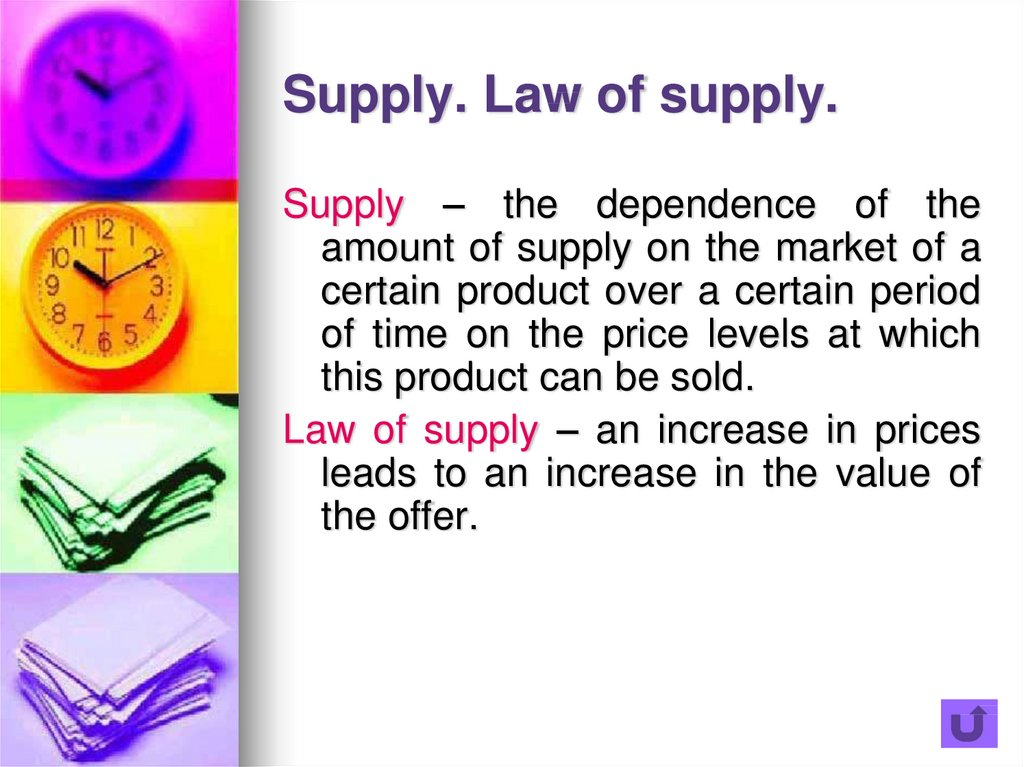# Supply and demand. Demand. Law of demand

## 1. Supply and demand

Magomedov Magomed 1-11

## 2. Demand. Law of demand.

Demand – the dependence of the
quantities of demand in a given
commodity market on the prices at
which goods can be offered for sale.
Law of demand– increasing prices
leads to a decrease in the amount of
demand.

## 3. Supply. Law of supply.

Supply – the dependence of the
amount of supply on the market of a
certain product over a certain period
of time on the price levels at which
this product can be sold.
Law of supply – an increase in prices
leads to an increase in the value of
the offer.

## 4. Non-price demand factors:

the tastes and preferences of
consumers
number of buyers on the market
prices for interchangeable and
complementary products
changes in consumer income
waiting for future price and
revenue changes

## 5. Change in demand

P
increase
decrease
О
Q
Influence of non-price factors

## 6. Influence of non-price factors

prices for production resources
technology
state taxes and subsidies
number of sellers on the market
prices of alternative products
expected future price changes for
this product

## 7. Изменение предложения

P
increase
decrease
О
Influence of non-price factors
Q

## 8. Равновесие на рынке.

P
Po
О
Po – equilibrium
price
Qo
Qo – equilibrium number
Q

## 9. Sample task № 1.

The demand for product A is
described by the equation:
Qd = 50 – 6 · Р.
Supply of goods А:
Qs = 4 · Р – 10.
Determine the equilibrium price
and quantity of the product А.

## 10. The demand for product A is described by the equation: Qd = 50-6 · P. The offer of the goods And: Qs = 4 · R – 10. Determine

the equilibrium price and quantity of product A.
At equilibrium:
Qd = Qs
50 – 6 · Р = 4 · Р – 10
10 · Р = 60
Р0 = 6
Q0 = 4 · 6 – 10 = 14
Answer: Р0 = 6, Q0 = 14.

## 11. Задача – образец № 2.

Use the available table data to build
supply and demand curves.
Determine the equilibrium price and
quantity using the graphical method.
Р,
rub.
5
20
Qd,
demand
8
2
Qs,
supply
2
5

Р
Р0 = 15
Q0 = 4
excess
20
deficit
5
2
5
8
Q

## 13. Выводы:

Возможные ситуации на рынке:
1. Balance
Qd = Qs
2. Deficit
Qd > Qs
3. Excess
Qd < Qs